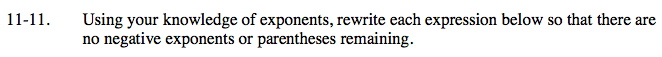### Home > CAAC > Chapter 11 > Lesson 11.1.1 > Problem11-11

11-11.Remember that to reduce fractional exponents, subtract the numerator from the denominator

$\frac{2}{4^2}$How many factors of s are multiplied?
How many of t?
How many of u?

$(s^{4}\cdot s^{7})(t\cdot t^{-1})(u^{2}) = S^{11}U^{2$Write (3w−2) four times and multiply them together following the same pattern as seen in part B.

$\frac{81}{W^2}$The square root of a number is the same as raising it to the 1/2 power

When you are multiplying two exponents together you add them.